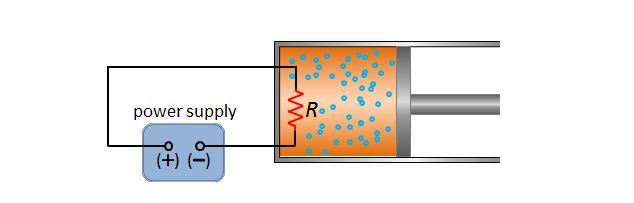# Ideal gas heatingThe above shows a resistor with resistance $R$ installed in a cylinder filled with a fixed quantity of ideal gas. We connect the resistor to a power supply with a voltage of $V$ to provide heating energy in the cylinder. Suppose the pressure and volume of the ideal gas before and after heating are $(P_0, V_0)$ and $(P_0, 1.5V_0),$ respectively. If the internal energy of the ideal gas before heating is $U_0,$ then what is the change in the amount of the internal energy $\Delta U$ after heating? (The cylinder is sealed completely.)

×